﻿ 网络拓扑特征的不平衡数据分类
«上一篇文章快速检索 高级检索

 智能系统学报2019, Vol. 14Issue (5): 889-896  DOI: 10.11992/tis.2018120140

### 引用本文PU Shiye, LIU Sanyang, BAI Yiguang. Imbalanced data classification of network topology characteristics[J]. CAAI Transactions on Intelligent Systems, 2019, 14(5): 889-896. DOI: 10.11992/tis.201812014.### 文章历史

Imbalanced data classification of network topology characteristics
PU Shiye, LIU Sanyang , BAI Yiguang
School of Mathematics and Statistics, Xidian University, Xi’an 710126, China
Abstract: This paper aims to solve the imbalanced data classification problem, which has been proven to be common in real applications. The dataset network structure is established to fully mine the topological features hidden outside the position information of sample points, analyze the connection characteristics of network nodes, and give these nodes different efficiencies. The similarity measure between the node to be tested and each sub-network is calculated, and the integrity measure between the node and each sub-network is further calculated according to the new probability model. A classification method of imbalanced data based on network topology features is constructed. An imbalanced factor c is introduced into the algorithm to reduce the influence caused by the difference in the number of positive and negative samples. The experimental results show that the algorithm can effectively improve the classification accuracy, especially for datasets with significant topological features. The classification performance and adaptability are further improved compared with the traditional classification method.
Key words: imbalanced data    similarity    network structure    accuracy rate    topology    physical feature

Silva等[8-9]将仅考虑样本点物理特征的传统分类方法视为低层次分类，把数据样本点看作网络节点，提出了基于网络信息特征的高层次数据分类方法，在训练样本点分类模型时既考虑了样本点的位置关系，又考虑到了数据点之间的拓扑特征，将两个层次的分类器有效地结合，并在数字图像识别中取得较高的准确度。Carnerio等提出了基于复杂网络的新型分类器，通过KNN法或KAOG法建立子网络模型，利用谷歌PageRank度量方法赋予网络节点不同影响力概念，依据Spatio structural effi-ciency和节点间的距离特征实现分类。文献针对复杂网络中的链路预测问题介绍了多种基于局部和全局结构的节点相似度模型，分析出实际复杂系统中网络节点的相互影响关系，两个节点之间产生连边的概率大小是由网络拓扑结构和几何结构共同决定的。文献中把链路预测问题视为一个二分类问题，提出了一个数据分类问题的概率模型，将待测样本点的类别归属于相似度分数高的类。

1 相关概念

1.1 节点局部效率

 ${p_i} = \left\{ {\begin{array}{l} {\delta ,}\quad{{D_{ i}} = 0} \\ {\frac{1}{{{D_{ i}}}}\sum\limits_{{e_{ ij}}} {{d_{ ij}},} }\quad{{D_{ i}} > 0} \end{array}} \right.$ (1)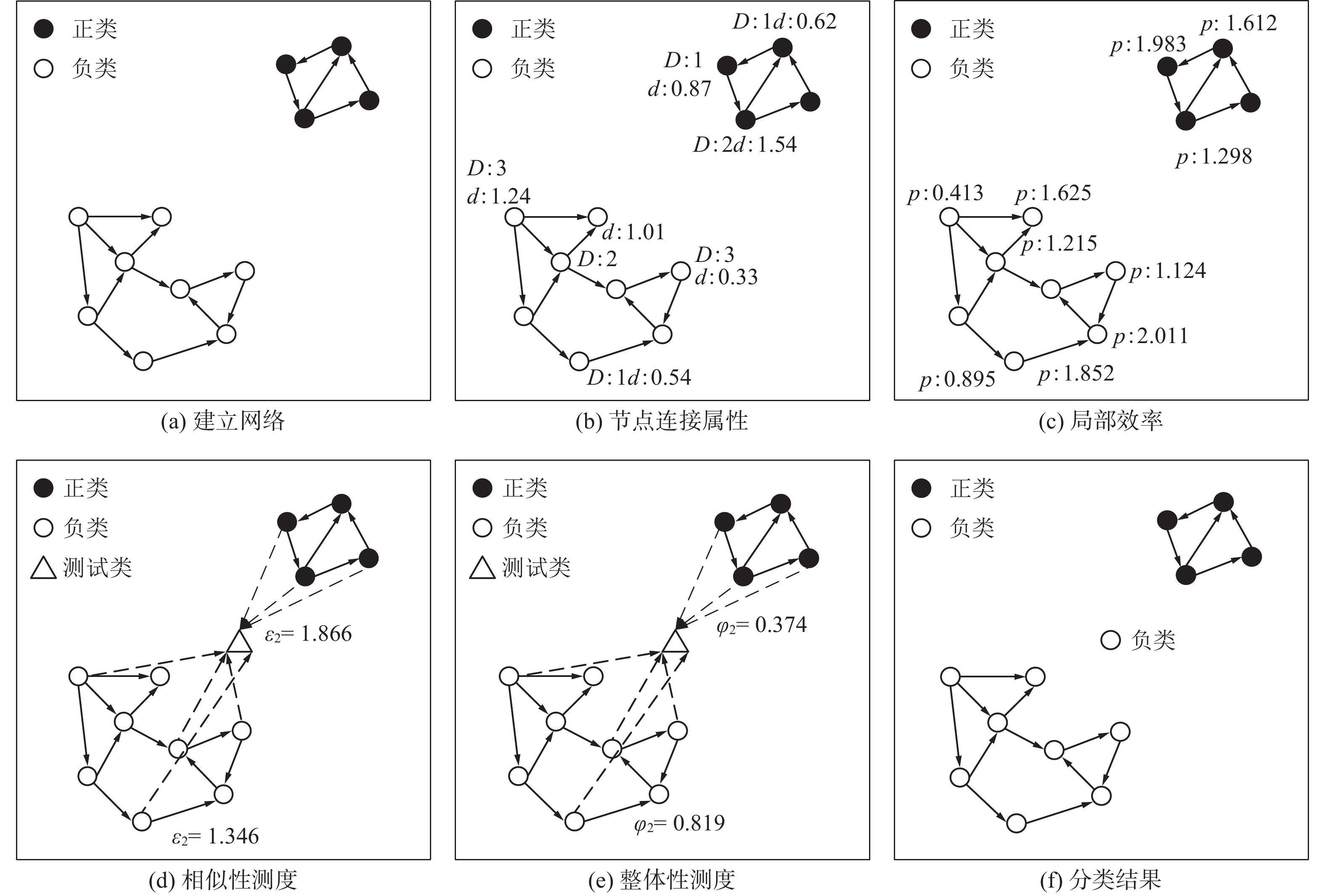Download: 图 1 NT-IDC的图解 Fig. 1 The diagram of NT-IDC
1.2 基于相似度的类别归属

 ${S_{i,j}} = \frac{1}{{D(i ,j)}}$

 $p({l_i}\left| u \right.) = \frac{{\sum\limits_{\{ v\left| {v \ne u} \right.,{\rm{Index}}(v) = {l_i}\} } \!\!\!\!\!\!\!\!\!\!\!\!\!\!\!\!\!{{S_{u,v}}} }}{{\sum\limits_{\{ v\left| {v \ne u} \right.,{\rm{Index}}(v) \ne 0\} } \!\!\!\!\!\!\!\!\!\!\!\!\!\!\!\!\!{{S_{u,v}}} }}$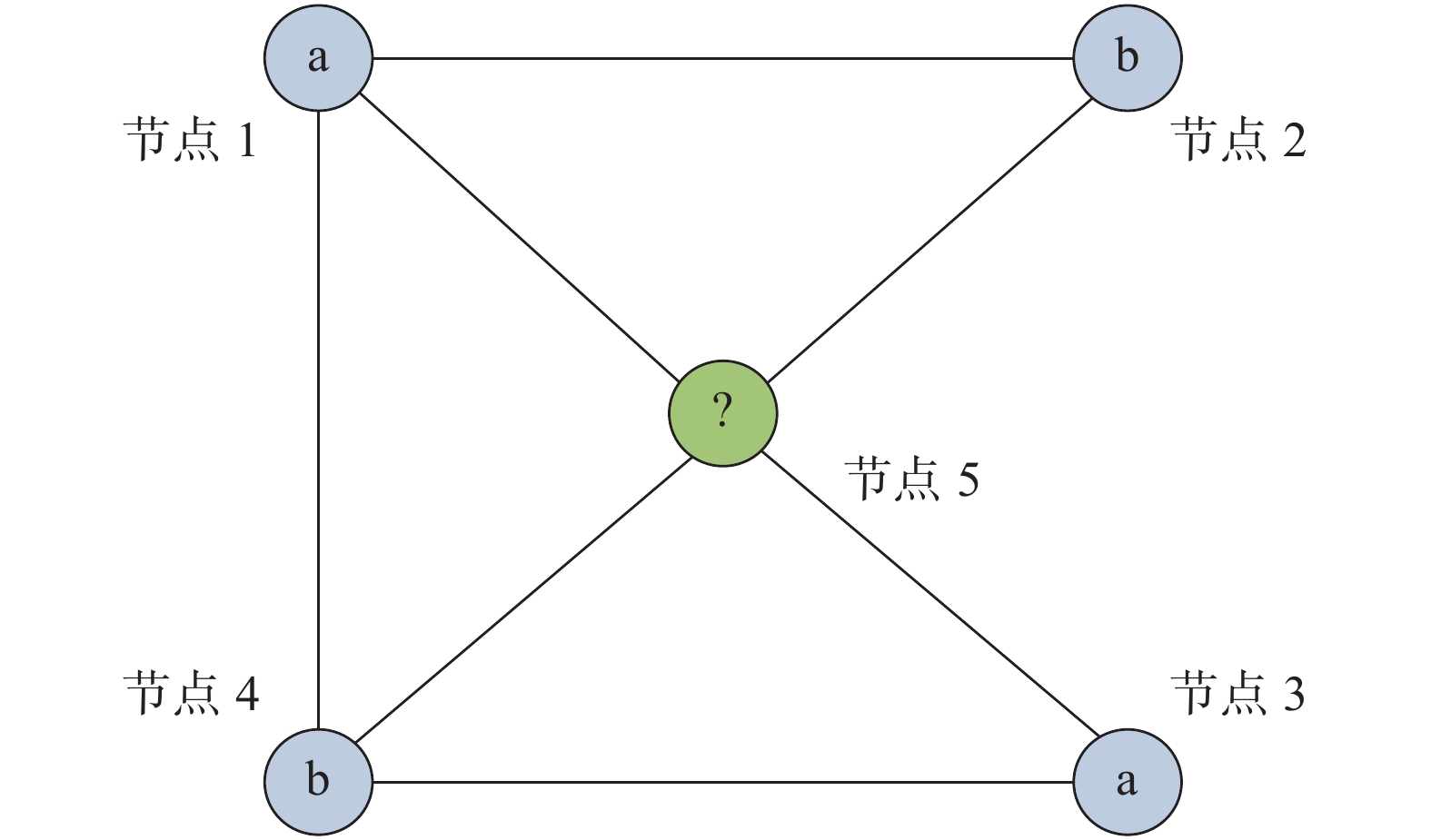Download: 图 2 预测节点的标签说明 Fig. 2 Description of the node label prediction
2 不平衡数据分类

2.1 相似性测度

 ${\varepsilon _{t n}} = \sum\limits_i {\frac{{{p_{ i}}}}{{{S_{ t,i}}}}}$ (2)

2.2 整体性测度

 ${\varphi _{t n}} = \frac{{{\varepsilon _{t n}} + c}}{{\sum\limits_{n = 1}^m {{\varepsilon _{t n}} + {\rho _{ n}} \cdot c} }}$ (3)

2.3 算法步骤和时间复杂度

1) 构建网络；

2) 根据式(1)计算网络节点局部效率；

3) 根据式(2)计算待测节点与每个子网络的相似性测度；

4) 根据式(3)计算待测节点与每个子网络的整体性测度；

5) 依据整体性测度的大小预测待测样本点的标签。

3 实验结果及分析 3.1 评价指标表 1 混淆矩阵 Tab.1 Confusion matrix

 $\rm{Se} = {\rm{TP}}/({\rm{TP}} + {\rm{FN}})$ (4)
 $\rm{Sp} = {\rm{TN}}/({\rm{FP}} + {\rm{TN}})$ (5)
 $\rm{Gm} = \sqrt {{\rm{Se}} \times {\rm{Sp}}}$ (6)

F-value是查全率Rc和敏感性Se的平均值， $\beta$ 是一个相对重要性参数，在不平衡数据分类问题中一般设置为1，查全率定义为 $\rm{Rc} = {\rm{TP}}/({\rm{TP}} + {\rm{FP}})$ ，则指标F-value为

 $\rm{F} - {\rm{value}} = \frac{{(1 + {\beta ^2}) \times {\rm{Rc}} \times {\rm{Se}}}}{{{\beta ^2} \times {\rm{Rc}} + {\rm{Se}}}}$ (7)
3.2 实验结果及分析

3.2.1 人造数据集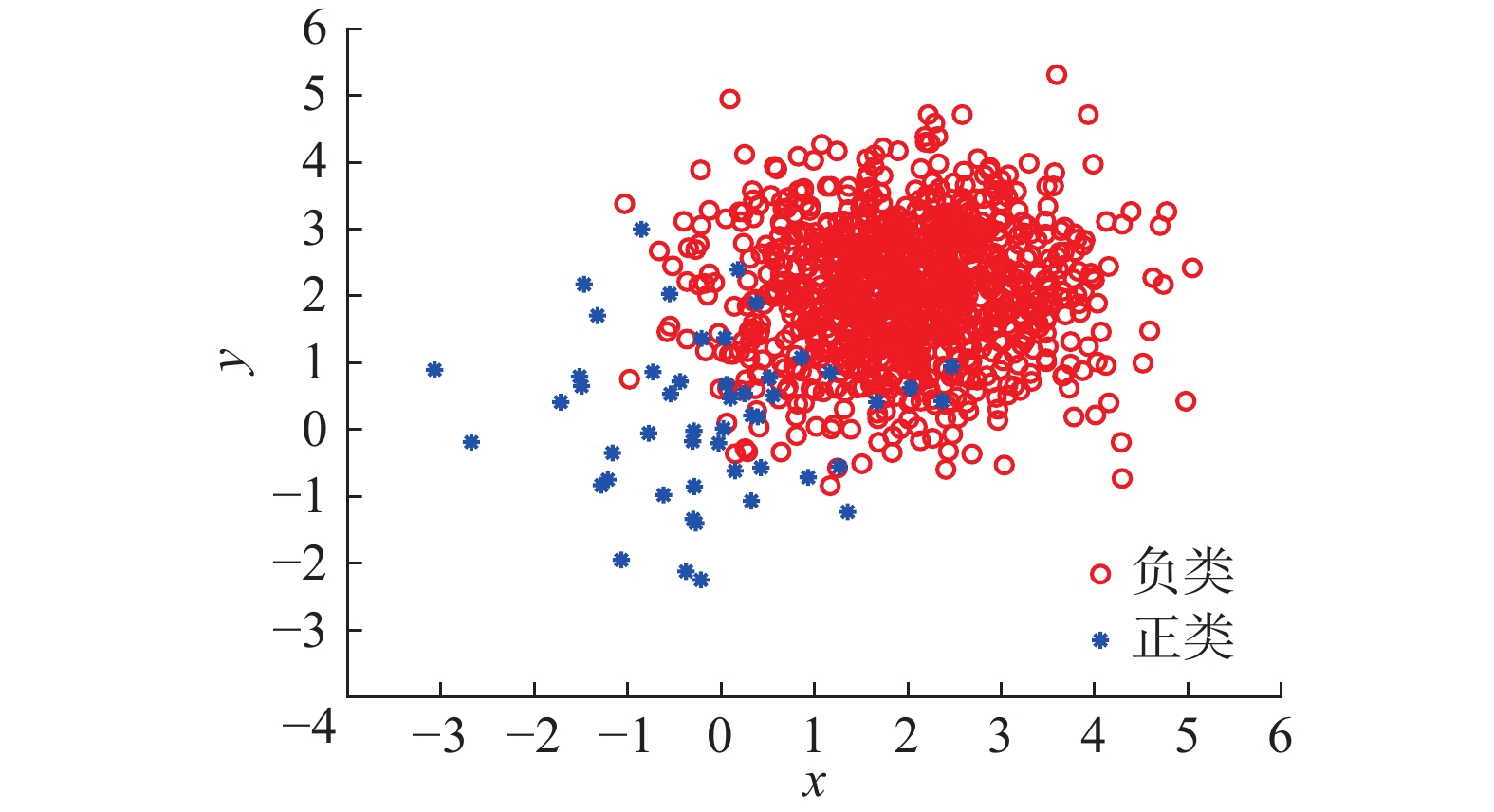Download: 图 3 人工数据集 Fig. 3 Artificial data set表 2 人工数据集的分类结果 Tab.2 The result of the artificial dataset
3.2.2 真实数据集表 3 数据集信息 Tab.3 Dataset information表 4 少数类分类结果 Tab.4 The classification result of minority class表 5 数据集在不同算法下的分类结果 Tab.5 The classification results of datasets under different algorithms

3.3 参数敏感性分析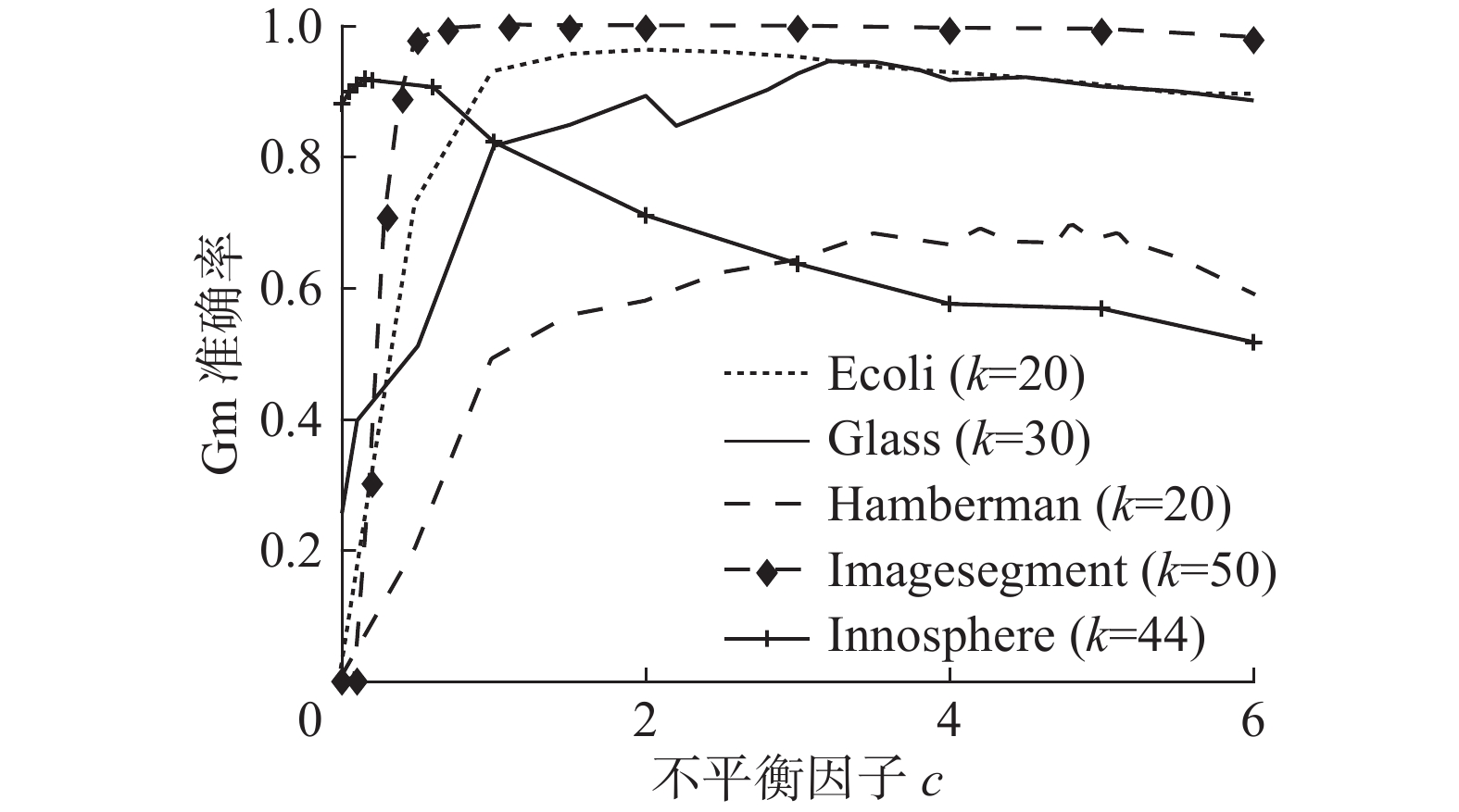Download: 图 4 参数 $c$ 对准确率Gm的影响 Fig. 4 The influence of parameter c on accuracy Gm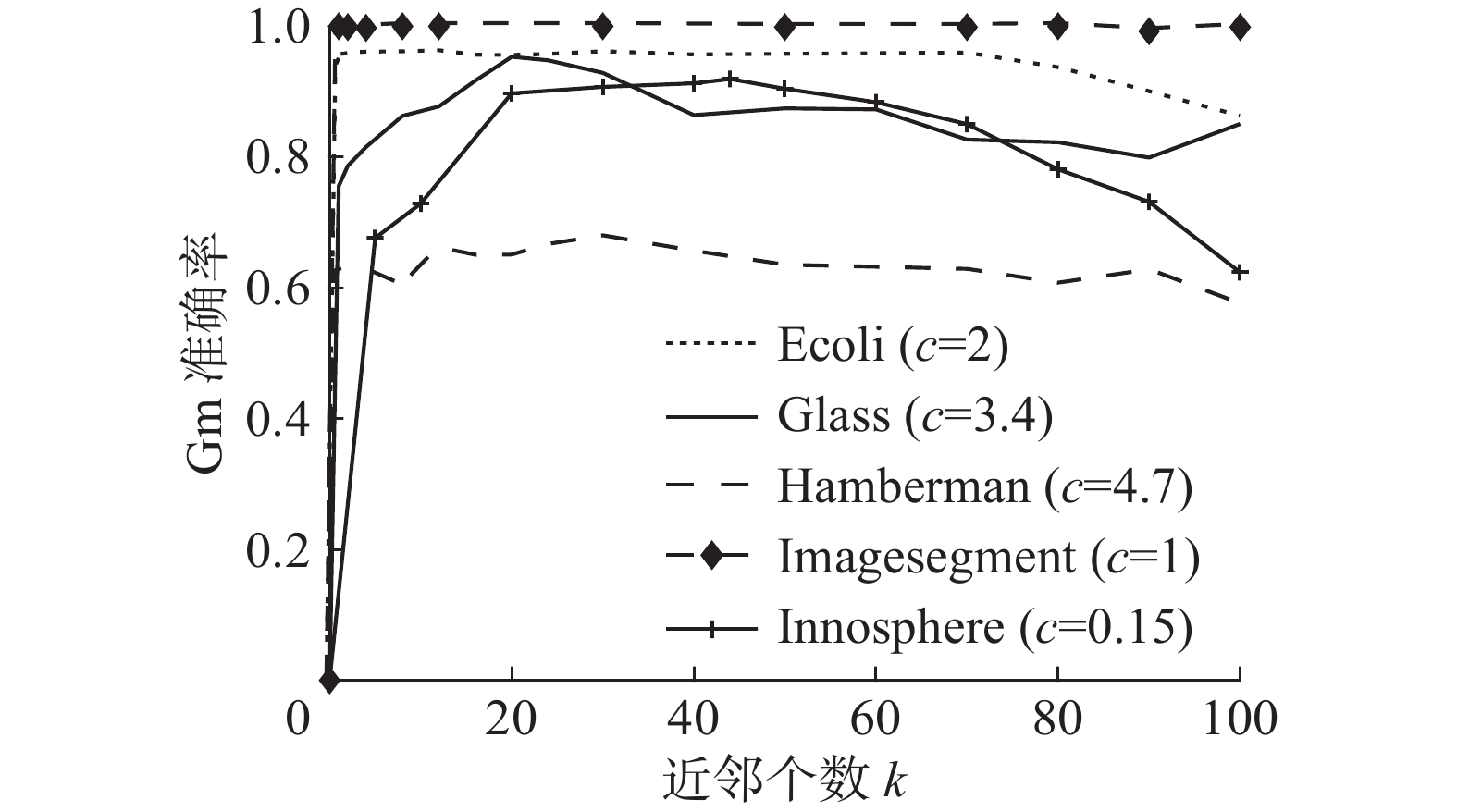Download: 图 5 参数 $k$ 对准确率Gm的影响 Fig. 5 The influence of parameter k on accuracy Gm

4 结束语

  HE Haibo, GARCIA E A. Learning from imbalanced data[J]. IEEE transactions on knowledge and data engineering, 2009, 21(9): 1263-1284. DOI:10.1109/TKDE.2008.239 (0)  KHOSHGOFTAAR T M, GOLAWALA M, VAN HULSE J. An empirical study of learning from imbalanced data using random forest[C]//Proceedings of the 19th IEEE International Conference on Tools with Artificial Intelligence. Patras, Greece, 2007: 310–317. (0)  LIN Chunfu, WANG Shengde. Fuzzy support vector machines[J]. IEEE transactions on neural networks, 2002, 13(2): 464-471. DOI:10.1109/72.991432 (0)  程险峰, 李军, 李雄飞. 一种基于欠采样的不平衡数据分类算法[J]. 计算机工程, 2011, 37(13): 147-149. CHENG Xianfeng, LI Jun, LI Xiongfei. Imbalanced data classification algorithm based on undersampling[J]. Computer engineering, 2011, 37(13): 147-149. DOI:10.3969/j.issn.1000-3428.2011.13.047 (0)  CHAWLA N V, BOWYER K W, HALL L O, et al. SMOTE: synthetic minority over-sampling technique[J]. Journal of artificial intelligence research, 2011, 16(1): 321-357. (0)  VEROPOULOS K, CAMPBELL I C G, CRISTIANINI N. Controlling the sensitivity of support vector machines[C]//Proceedings of the International Joint Conference on Artificial Intelligence. Stockholm, Sweden, 1999: 55–60. (0)  张银峰, 郭华平, 职为梅, 等. 一种面向不平衡数据分类的组合剪枝方法[J]. 计算机工程, 2014, 40(6): 157-161, 165. ZHANG Yinfeng, GUO Huaping, ZHI Weimei, et al. An ensemble pruning method for imbalanced data classification[J]. Computer engineering, 2014, 40(6): 157-161, 165. DOI:10.3778/j.issn.1002-8331.1204-0414 (0)  SILVA T C, ZHAO Liang. Network-based high level data classification[J]. IEEE transactions on neural networks and learning systems, 2012, 23(6): 954-970. DOI:10.1109/TNNLS.2012.2195027 (0)  SILVA T C, ZHAO Liang. High-level pattern-based classification via tourist walks in networks[J]. Information sciences, 2015, 294: 109-126. DOI:10.1016/j.ins.2014.09.048 (0)  CARNEIRO M G, ZHAO Liang. Organizational data classification based on the importance concept of complex networks[J]. IEEE transactions on neural networks and learning systems, 2018, 29(8): 3361-3373. DOI:10.1109/TNNLS.2017.2726082 (0)  BERTINI JR J R, ZHAO Liang, MOTTA R, et al. A nonparametric classification method based on K-associated graphs [J]. Information sciences, 2011, 181(24): 5435-5456. DOI:10.1016/j.ins.2011.07.043 (0)  LÜ Linyuan, ZHOU Tao. Link prediction in complex networks: A survey[J]. Physical A: statistical mechanics and its applications, 2011, 390(6): 1150-1170. DOI:10.1016/j.physa.2010.11.027 (0)  ZHANG Qianming, SHANG Mingsheng, LÜ Linyuan. Similarity-based classification in partially Labeled networks[J]. International journal of modern physical C, 2010, 21(6): 813-824. DOI:10.1142/S012918311001549X (0)  BIRX D L, PIPENBERG S J. A complex mapping network for phase sensitive classification[J]. IEEE transactions on neural networks, 1993, 4(1): 127-135. DOI:10.1109/72.182703 (0)  WANG Meng, FU Weijie, HAO Shijie, et al. Learning on big graph: label inference and regularization with anchor hierarchy[J]. IEEE transactions on knowledge and data engineering, 2017, 29(5): 1101-1114. DOI:10.1109/TKDE.2017.2654445 (0)  CONG Chen, LIU Tongliang, TAO Dacheng, et al. Deformed graph laplacian for semisupervised learning[J]. IEEE transactions on neural networks and learning systems, 2015, 26(10): 2261-2274. DOI:10.1109/TNNLS.2014.2376936 (0)  顾苏杭, 王士同. 基于数据点本身及其位置关系辅助信息挖掘的分类方法[J]. 模式识别与人工智能, 2018, 31(3): 197-207. GU Suhang, WANG Shitong. Classification approach by mining betweenness information beyond data points themselves[J]. Pattern recognition and artificial intelligence, 2018, 31(3): 197-207. (0)  TSANG I W H, KWOK J T Y, ZURADA J M. Generalized core vector machines[J]. IEEE transactions on neural networks, 2006, 17(5): 1126-1140. DOI:10.1109/TNN.2006.878123 (0)  赵自翔, 王广亮, 李晓东. 基于支持向量机的不平衡数据分类的改进欠采样方法[J]. 中山大学学报(自然科学版), 2012, 51(6): 10-16. ZHAO Zixiang, WANG Guangliang, LI Xiaodong. An improved SVM based under-sampling method for classifying imbalanced data[J]. Acta Scientiarum Naturalium Universitatis Sunyatseni, 2012, 51(6): 10-16. (0)